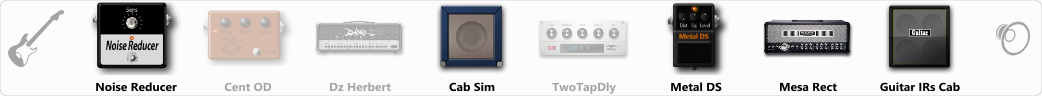# COBAIN PRESET

Discussion in 'ToneLib-GFX presets' started by lilian, Oct 11, 2020.

1. COBAIN PRESET

Preset name: Cobain preset

Effects chain:Effect: "Noise Reducer" (Dynamics / Filter), active - "yes"
"Sens" = 50
"Mode" = Hard

Effect: "Cent OD" (Overdrive / Distortion), active - "no"
"Drive" = 96
"Tone" = 77
"Level" = 38

Effect: "Dz Herbert" (Amp simulators), active - "no"
"Gain" = 46
"Bass" = 50
"Middle" = 54
"Treble" = 60
"Presence" = 87
"Master" = 100
"Output" = 53
"Level (dB)" = 0

Effect: "Cab Sim" (Cabinets), active - "yes"
"Model" = 2x12" Silver Bell
"Level (dB)" = 0

Effect: "TwoTapDly" (Delay), active - "no"
"Time" = 756
"Feedback" = 44
"Tone" = 77
"Sens" = 0
"TapTime" = 5.0
"Mix" = 60

Effect: "Metal DS" (Overdrive / Distortion), active - "yes"
"Dist" = 100
"Bass" = 74
"Middle" = 60
"Treble" = 46
"Level" = 57

Effect: "Mesa Rect" (Amp simulators), active - "yes"
"Gain" = 50
"Bass" = 43
"Middle" = 61
"Treble" = 60
"Presence" = 84
"Master" = 50
"Output" = 50
"Level (dB)" = 0

Effect: "Guitar IRs Cab" (Cabinets), active - "yes"
"Model" = Mesa Rectifier (4x12")
"Mic Position" = Center
"Mic Distance" = Middle
"Low Cut (Hz)" = 152
"Hi Cut (kHz)" = 12.5
"Mix" = 100
"Level (dB)" = 6

Note: You will need to download and install the ToneLib-GFX software to use the preset.

#### Attached Files:

• ###### COBAIN_PRESET.tlgfx
File size:
678 bytes
Views:
2,472
Lizzzard___, diegolol and oriten3 like this.